NEET  >  Simple Harmonic Motion

# Simple Harmonic Motion - Physics Class 11 - NEET

Harmonic Oscillation:

The oscillation which can be expressed in terms of single harmonic function, i.e., sine or cosine function, is called harmonic oscillation.

Simple Harmonic Motion:

A harmonic oscillation of constant amplitude and of single frequency under a restoring force whose magnitude is proportional to the displacement and always acts towards mean Position is called Simple Harmonic Motion (SHM).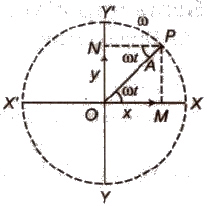A simple harmonic oscillation can be expressed as

y = a sin ωt

or y = a cos ωt

Where a = amplitude of oscillation.

Non-harmonic Oscillation:

A non-harmonic oscillation is a combination of two or more than two harmonic oscillations.

It can be expressed as y = a sin ωt + b sin 2ωt

Some Terms Related to SHM:

(i) Time Period: Time taken by the body to complete one oscillation is known as time period. It is denoted by T.

(ii) Frequency: The number of oscillations completed by the body in one second is called frequency. It is denoted by v.

Its SI unit is ‘hertz’ or ‘second-1‘.

Frequency = 1 / Time period

(iii) Angular Frequency: The product of frequency with factor 2π is called angular frequency. It is denoted by ω.

Angular frequency (ω) = 2πv

Its SI unit is ‘hertz’ or ‘second-1‘.

(iv) Displacement: A physical quantity which changes uniformly with time in a periodic motion is called displacement. It is denoted by y.

(v) Amplitude: The maximum displacement in any direction from mean position is called amplitude. It is denoted by a.

(vi) Phase: A physical quantity which express the position and direction of motion of an oscillating particle, is called phase. It is denoted by φ.

Simple harmonic motion is defined as the projection of a uniform circular motion on any diameter of a circle of reference.

Some Important Formula of SHM:

(i) Displacement in SHM at any instant is given by

y = a sin ωt

or y = a cos ωt

where a = amplitude and

ω = angular frequency.

(ii) Velocity of a particle executing SHM at any instant is given by

v = ω √(a2 – y2)

At mean position y = 0 and v is maximum

vmax = aω

At extreme position y = a and v is zero.

(iii) Acceleration of a particle executing SHM at any instant is given by

A or α = – ω2 y

Negative sign indicates that the direction of acceleration is opposite to the direction in which displacement increases, i.e., towards mean position.

At mean position y = 0 and acceleration is also zero.

At extreme position y = a and acceleration is maximum

Amax = – aω2

(iv) Time period in SHM is given by

T = 2π √Displacement / Acceleration

Graphical Representation:

(i) Displacement – Time Graph: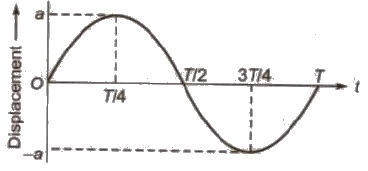(ii) Velocity – Time Graph: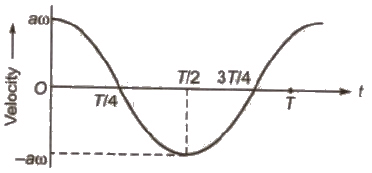(iii) Acceleration – Time Graph: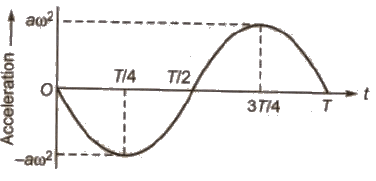Note The acceleration is maximum at a place where the velocity is minimum and vice – versa.

For a particle executing SHM, the phase difference between

(i) Instantaneous displacement and instantaneous velocity

(ii) Instantaneous velocity and instantaneous acceleration

(iii) Instantaneous acceleration and instantaneous displacement

The graph between velocity and displacement for a particle executing SHM is elliptical.

Force in SHM:

We know that, the acceleration of body in SHM is α = -ω2 x

Applying the equation of motion F = ma,

We have, F = – mω2 x = -kx

Where, ω = √k / m and k = mω2 is a constant and sometimes it is called the elastic constant.

In SHM, the force is proportional and opposite to the displacement.

Energy in SHM:

The kinetic energy of the particle is K = 1 / 2 mω2 (A2 – x2)

From this expression we can see that, the kinetic energy is maximum at the centre (x = 0) and zero at the extremes of oscillation (x ± A).

The potential energy of the particle is U = 1 / 2 mω2 x2

From this expression we can see that, the potential energy has a minimum value at the centre (x = 0) and increases as the particle approaches either extreme of the oscillation (x ± A).

Total energy can be obtained by adding potential and kinetic energies. Therefore,

E = K + U

= 1 / 2 mω2 (A2 – x2) + 1 / 2 mω2 x2

= 1 / 2 mω2 A2

where A = amplitude

m = mass of particle executing SHM.

ω = angular frequency and

v = frequency

Changes of kinetic and potential energies during oscillations.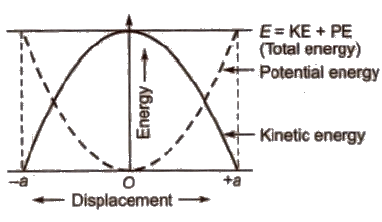The frequency of kinetic energy or potential energy of a particle executing SHM is double than that of the frequency in SHM.

The frequency of total energy of particles executing SHM is zero as total energy in SHM remains constant at all positions.

When a particle of mass m executes SHM with a constant angular frequency (I), then time period of oscillation

T = 2π √Inertia factor / Spring factor

In general, inertia factor = m, (mass of the particle)

Spring factor = k (force constant)

How the different physical quantities (e.g., displacement, velocity, acceleration, kinetic energy etc) vary with time or displacement are listed ahead in tabular form.

 S.No. Name of the equation Expression of the equation Remarks 1. Displacement-time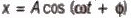x varies between + A and - A 2. Velocity-time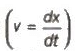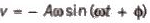v varies between + Aω and - A ω 3. Acceleration-time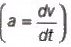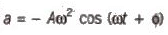a varies between + Aω2 and - Aω2 4. Kinetic energy-time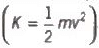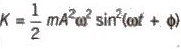K varies between and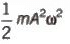5. Potential energy-time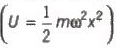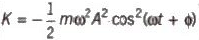U varies between and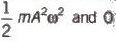6. Total energy-time(E = K + U)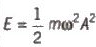E is constant 7. Velocity-displacement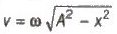v = 0 at x = ±4 and at x = 0 , v = ± Aω 8. Acceleration-displacement a = - ω2X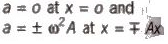9. Kinetic energy-displacement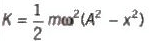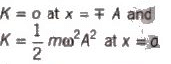10. Potential energy-d displacement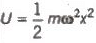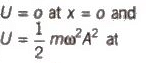11. Total energy-d displacement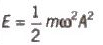x = ± A E is constant

Oscillations of Liquid in a U – tube:

If a liquid is filled up to height h in both limbs of a U-tube and now liquid is depressed upto a small distance y in one limb and then released, then liquid column in U-tube start executing SHM.

The time period of oscillation is given by

T = 2π √h / g

Oscillations of a floating cylinder in liquid is given by

T = 2π √l / g

where I = length of the cylinder submerged in liquid in equilibrium.

When a spring is compressed or stretched through a small distance y from mean position, a restoring force acts on it.

Restoring force (F) = – ky

where k = force constant of spring.

If a mass m is suspended from a spring then in equilibrium,

mg = kl

This is also called Hooke’s law.

Time period of a loaded spring is given by:

T = 2π √m / k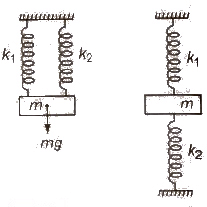When two springs of force constants k1 and k2 are connected in parallel to mass m as shown in figure, then

(i) Effective force constant of the spring combination

k = k1 + k2

(ii) Time period T = 2π √m / (k1 + k2)

When two springs of force constant k1 and k2 are connected in series to mass m as shown in figure, then

(i) Effective force constant of the spring combination

1 / k = 1 / k1 + 1 / k2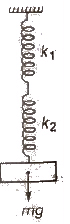(ii) Time period T = 2π √m(k1 + k2) / k1k2

Free Oscillations:

When a body which can oscillate about its mean position is displaced from mean position and then released, it oscillates about its mean position. These oscillations are called free oscillations and the frequency of oscillations is called natural frequency.

The document Simple Harmonic Motion | Physics Class 11 - NEET is a part of the NEET Course Physics Class 11.
All you need of NEET at this link: NEET

## FAQs on Simple Harmonic Motion - Physics Class 11 - NEET

 1. What is simple harmonic motion?Ans. Simple harmonic motion is a type of periodic motion where an object oscillates back and forth around a stable equilibrium position. It occurs when the restoring force acting on the object is directly proportional to its displacement from the equilibrium position, and the motion is sinusoidal.
 2. How is the period of simple harmonic motion related to its frequency?Ans. The period of simple harmonic motion is the time taken for one complete oscillation, while the frequency is the number of oscillations per unit time. They are inversely related to each other: the period is equal to the reciprocal of the frequency, and vice versa.
 3. What is the amplitude of simple harmonic motion?Ans. The amplitude of simple harmonic motion is the maximum displacement of the object from its equilibrium position. It represents the maximum distance the object moves away from its equilibrium and indicates the maximum potential energy stored in the system.
 4. How does the mass of an object affect its simple harmonic motion?Ans. The mass of an object does not affect the period or frequency of simple harmonic motion. In a simple harmonic oscillator, such as a mass-spring system, the period and frequency depend only on the stiffness of the spring and the object's restoring force, not its mass.
 5. What is the relationship between simple harmonic motion and Hooke's Law?Ans. Simple harmonic motion is closely related to Hooke's Law, which states that the force exerted by a spring is directly proportional to the displacement from its equilibrium position. Hooke's Law provides the restoring force that drives the oscillatory motion in simple harmonic systems, such as mass-spring systems.

## Physics Class 11

130 videos|483 docs|210 tests

## Physics Class 11

130 videos|483 docs|210 tests

### How to Prepare for NEET

Read our guide to prepare for NEET which is created by Toppers & the best Teachers
Signup to see your scores go up within 7 days! Learn & Practice with 1000+ FREE Notes, Videos & Tests.
10M+ students study on EduRev
Track your progress, build streaks, highlight & save important lessons and more!(Scan QR code)
Related Searches

,

,

,

,

,

,

,

,

,

,

,

,

,

,

,

,

,

,

,

,

,

;